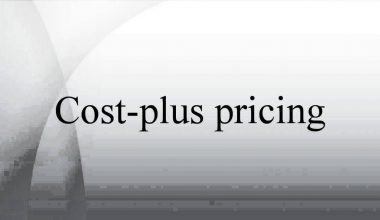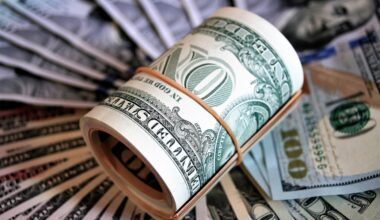# Rate of Return (ROR): How to Calculate Rate of Return

If you wish to invest in a financial market or firm, you must first grasp what the rate of return is. In this post, you will discover what rate of return and the annual rate of return is, how to calculate it, the formula, examples and how to analyze your financial returns in various ways.

## What is the Rate of Return?

A Rate of Return (ROR) is the gain or loss of an investment over a certain time period. In other words, the ROR is the difference between the gain (or loss) and the cost of the initial investment, often stated as a percentage. When the ROR is positive, the investment has made a profit; when the ROR is negative, the investment has lost money.

## Rate of Return Formula

The following is the typical formula for determining ROR:

Keep in mind that any gains made throughout the investment’s holding period should be factored into the formula. For example, if a share costs \$10 and its current price is \$15, with a \$1 dividend received throughout the time, the dividend should be factored into the ROR formula. It is calculated as follows:

((\$15 + \$1 – \$10) / \$10) x 100 = 60%

## Example of How to Calculate Rate of Return

Roy is a retail investor who decides to buy 10 shares of Company A at \$20 per unit. Roy holds Company A stock for two years. During that time period, Company A paid annual dividends of \$1 per share. After two years, Roy chooses to sell all ten shares of Company A at the ex-dividend price of \$25. Roy wants to calculate what his rate of return was for the two years he owned the shares.
To calculate the rate of return, the first total the dividends he earned throughout the two-year period:

Dividends from 10 shares = \$20 × (\$1 annual dividend x 2)

Next, calculate out how much he sold the shares for:

10 shares multiplied by \$25 is \$250. (Gain from selling 10 shares)

Finally, calculate how much it cost Roy to buy 10 shares of Company A:

10 x \$20 = \$200 (Cost of purchasing 10 shares)

Enter the following figures into the rate of return formula:

((\$250 + \$20 – \$200) / \$200) x 100 = 35%

As a result, Roy earned a 35% return on his investment over the course of two years.

## Annual Rate of Return

It is important to note that the regular ROR reflects the gain or loss of an investment over an arbitrary time period, given as a percentage. The annual rate of return, commonly known as the Compound Annual Growth Rate (CAGR), is the annualized return on investment.

### Annual Rate of Return Formula

The annualized rate of return formula is as follows:

Any gains generated during the holding period of this investment, similar to the simple ROR, should be included in the formula.

### Annual Rate of Return Example

Let’s go back to the previous example and calculate the annualized rate of return. Remember that Adam bought 10 shares for \$20 each unit, earned \$1 in dividends per share each year, then sold the shares for \$25 after two years. The annualized ROR is as follows:

((\$250 + \$20) / \$200 )1/2 – 1 = 16.1895%

As a result, Roy’s investment yielded an annualized return of 16.1895 percent.

## Alternative Return Measures

Return can mean different things to different people, and understanding what they mean requires understanding the context of the scenario. In addition to the methods described above for calculating returns, there are various sorts of formulas.

Alternative measures of return that are commonly used include:

• Return on Equity (ROE)
• Return on Assets (ROA)
• Internal Rate of Return (IRR)
• Return on Investment (ROI)
• Return on Invested Capital (ROIC)

## Rate and Bond Rates of Return (RoR)

The ROR calculation for equities and bonds differs slightly. Assume an investor pays \$60 per share for a stock, holds it for five years, and earns a total of \$10 in dividends. If the investor sells the stock for \$80, his per-share gain is \$80 minus \$60, which equals \$20. He has also received \$10 in dividend income, for a total gain of \$20 + \$10 = \$30. The stock’s ROR is thus 50%, meaning a \$30 gain per share divided by a \$60 cost per share.

Consider the case of an investor who pays \$1,000 for a \$1,000 par value 5% coupon bond. Each year, the investment yields \$50 in interest income. If the investor sells the bond for \$1,100 in premium value and receives \$100 in total interest, the investor’s ROR is equal to the \$100 gain on sale plus the \$100 interest income divided by the \$1,000 initial cost, or 20%.

## Nominal Rate of Return (NRR) vs. Rate of Return (RoR)

Because it does not account for the effect of inflation over time, the simple ROR is termed a nominal rate of return. Inflation diminishes the purchasing power of money, thus \$335,000 today is not the same as \$335,000 six years from now.

One method of accounting for the time value of money is discounting. When the effect of inflation is factored in, we refer to this as the actual ROR (or the inflation-adjusted rate of return).

## Compound Annual Growth Rate (CAGR) vs. Real Rate of Return (RoR)

The compound annual growth rate (CAGR) is a notion that is closely related to the simple ROR. The CAGR is the average annual rate of return on an investment over a time period of more than one year, implying that the computation must account for growth over various time periods.

To calculate compound annual growth rate, divide the value of an investment at the end of the period in question by its value at the start of the period in question; raise the result to the power of one divided by the number of holding periods, such as years; and subtract one from the resulting result.

## Internal Rate of Return (IRR) and Discounted Cash Flow (DCF)

The next step in comprehending RoR over time is to account for the time value of money (TVM), which the CAGR does not take into account. Discounted cash flows take an investment’s earnings and discount each cash flow depending on a discount rate. The discount rate is the investor’s accepted minimum ROR or the projected rate of inflation. Businesses, like investors, use discounted cash flows to evaluate the profitability of their investments.

Assume, for example, that a company is considering purchasing a new piece of equipment for \$10,000 at a discount rate of 5%. Following a \$10,000 cash expenditure, the equipment is utilized in the business’s activities, increasing cash inflows by \$2,000 each year for the next five years. For the next five years, the company will apply present value table factors to the \$10,000 outflow and the \$2,000 inflow.

The \$2,000 influx in year five would be discounted using a five-year discount rate of 5%. The investment is profitable if the sum of all adjusted cash inflows and outflows is greater than zero. A positive net cash inflow indicates that the ROR is greater than the 5% discount rate.

The internal rate of return is another name for the ROR utilizing discounted cash flows (IRR). The internal rate of return (IRR) is a discount rate that equalizes the net present value (NPV) of all cash flows from a certain project or investment. IRR estimates use the same formula as NPV and the time value of money (using interest rates).

## What is a good rate of return?

A good return on investment is generally thought to be around 7% per year.

## What is a good rate of return on 401k?

According to many retirement advisers, the average 401(k) portfolio generates an annual return of 5% to 8%, depending on market conditions.

## What is the average stock market return over 10 years?

According to Goldman Sachs data, the average 10-year stock market return is 9.2 percent. The S&P 500 index has done somewhat better, returning 13.6 percent each year. The average return varies greatly from year to year, however, maintaining investments over time can assist.

## What is the average annual return?

The average annual return (AAR) is a percentage used when reporting historical returns, such as a mutual fund’s three-, five-, and ten-year average returns. In its most basic form, the average annual return (AAR) measures how much money a mutual fund makes or loses over a specific time period.

## How do I increase my rate of return?

Boost your sales and revenues, or raise your prices, to increase your return on investment. You’ve enhanced your return if you can expand sales and revenues without increasing costs, or just increase costs enough to give a net profit gain.

## Is a high required rate of return good?

If the needed rate of return is too high, it effectively steers investment behavior toward riskier ventures.

##### You May Also Like## CONDO VS COOP: Understanding the Differences

Table of Contents Hide What Is a Condo?What Is a Coop?Understanding Condo vs Coop Differences and Investment The…## COST PLUS PRICING: Meaning, Example, Advantages & Disadvantages

Table of Contents Hide What is Cost plus pricing?Cost Plus Pricing AnalysisCost plus pricing ExampleAdvantages of cost plus…## Active Investing vs. Passive: Understanding Why You Need Both (+Detailed Guide)

Table of Contents Hide Active vs. Passive Investing: An OverviewActive Investing Passive InvestingActive vs Passive Investing: Understanding its…## ROBINHOOD REVIEW: Why Robinhood is Bad (+ Detailed guide)